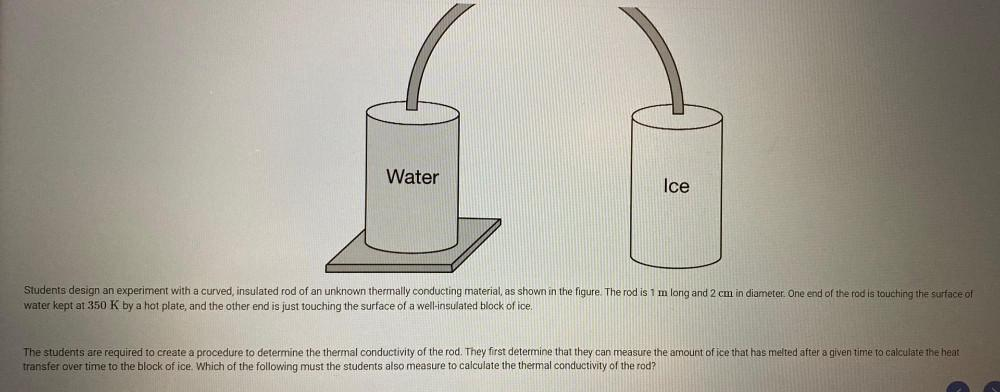Question:

# Students design an experiment with a curved, insulated rod of an unknown thermally conducting material, as shown in the figure. The rod is 1m long and 2 cm in diameter. One end of the rod is touchingStudents design an experiment with a curved, insulated rod of an unknown thermally conducting material, as shown in the figure. The rod is 1m long and 2 cm in diameter. One end of the rod is touching the surface of water kept at 350 K by a hot plate, and the other end is just touching the surface of a well-insulated block of ice. The students are required to create a procedure to determine the thermal conductivity of the rod. They first determine that they can measure the amount of ice that has melted after a given time to calculate the heat transfer over time to the block of ice. Which of the following must the students also measure to calculate the thermal conductivity of the rod?